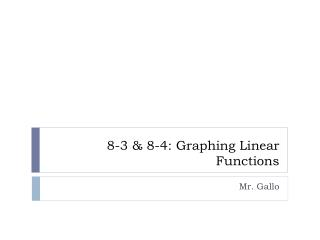DownloadDownload Presentation8-3 & 8-4: Graphing Linear Functions

# 8-3 & 8-4: Graphing Linear Functions

Download Presentation## 8-3 & 8-4: Graphing Linear Functions

- - - - - - - - - - - - - - - - - - - - - - - - - - - E N D - - - - - - - - - - - - - - - - - - - - - - - - - - -
##### Presentation Transcript

1. 8-3 & 8-4: Graphing Linear Functions Mr. Gallo

2. Graphing Linear Functions • Linear Function: • The graph of this function is a____________ _______. • Graphs of the form y = mx line straight

3. Graphing Linear Functions • Example 1: Given the point A (3, 6), find the equation of the line that passes through A and the origin. 3 What is the slope of the line? ______________________What could you multiply by 3 to get 6? _______________________The equation of the line is: y= x 6

4. Graphing Linear Functions • Example 2-Given the point B (9, 3), find the equation of the line that passes through B and the origin. What is the slope of the line? ______________________ What could you multiply by 9 to get 3? ______________________ The equation of the line is: y = x 9 3

5. The Slope Intercept Form • The equation of line in the slope-intercept form: • represents the _________. • represents the _______________. • y-intercept is where the line crosses the y-axis slope y -intercept

6. Example 3 - Graph the equation What is the y-intercept? _______ What is the slope? ______ Example 4 - Graph the equation What is the y-intercept? ______ What is the slope? ______

7. How are the graphs alike? ________________________________________________________________________ They have the same slopes. Parallel lines How are they different? ____________________________________________________________________ They have different y –intercepts.

8. Example 5- Find the equation of the line with a y-intercept of 1 and a slope 3. Equation: _____________

9. Example 6- Find the equation of the line passing through (0, 6) and with slope −4. Slope: _________. Substitute Point: Equation: _____________

10. Example 7: Find the equation for the line passing through (3, −4) and (9, 0). Slope: _________. Substitute Point: Equation: _____________

11. Homework: 8.3-8.4 Worksheet